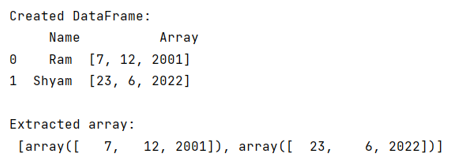# How to extract NumPy arrays from specific column in pandas frame and stack them as a single NumPy array?

Given a NumPy array, we have to extract from specific column in pandas frame and stack them as a single NumPy array. By Pranit Sharma Last updated : September 23, 2023

Pandas is a special tool that allows us to perform complex manipulations of data effectively and efficiently. Inside pandas, we mainly deal with a dataset in the form of DataFrame. DataFrames are 2-dimensional data structures in pandas. DataFrames consist of rows, columns, and the data. The Data inside the DataFrame can be of any type.

On the other hand, NumPy is an abbreviated form of Numerical Python. It is used for different types of scientific operations in Python.

NumPy is a vast python library used for almost every kind of scientific or mathematical operation. It is itself an array that is a collection of various methods and functions for processing the arrays.

## Problem statement

Given a NumPy array, we have to extract from specific column in pandas frame and stack them as a single NumPy array.

## Extracting NumPy arrays from specific column in pandas frame

To extract a NumPy array from a pandas DataFrame and convert them into a single NumPy array. This is an easy task in pandas as it provides us .tolist() method which will convert the values of a particular column into a NumPy array.

Note

To work with pandas, we need to import pandas package first, below is the syntax:

```import pandas as pd
```

Let us understand with the help of an example,

## Python code to extract NumPy arrays from specific column in pandas frame and stack them as a single NumPy array

```# Importing pandas package
import pandas as pd

# Importing numpy package
import numpy as np

# Creating a dictionary
d = {
'Name':['Ram','Shyam'],
'Array':[np.array([7,12,2001]),np.array([23,6,2022])]
}

# Creating dataframe
df = pd.DataFrame(d)

# Display DataFrame
print("Created DataFrame:\n",df,"\n")

# Extracting specific column and making it an array
result = df['Array'].tolist()

# Display result
print("Extracted array:\n",result)
```

### Output

The output of the above program is: